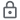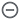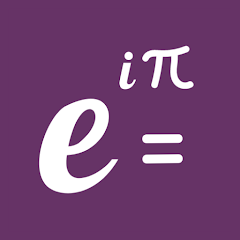# Math Factor - Equation Solver

100+Everyone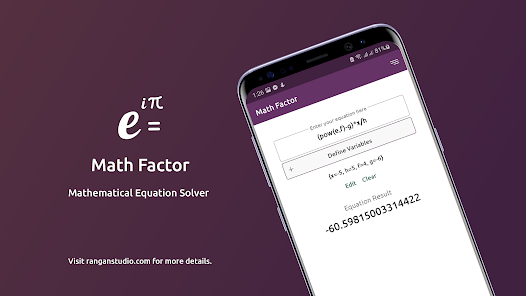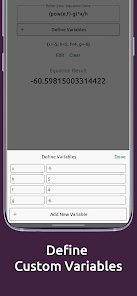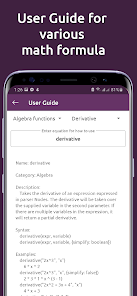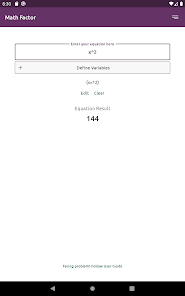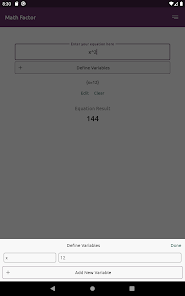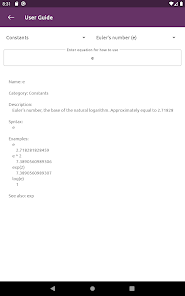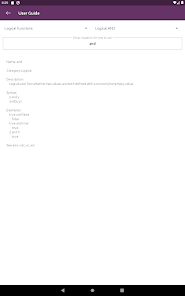Math Factor is an equation solver app where you can have solutions for thousands of mathematics formula, applications, examples, useful mathematical constants, usages, unit conversions and more.

The app contains a user guide to help you execute all the mathematical equations in scientific or computer readable format where the app will give the result with fastest computations. You can also define custom variables for each expressions.

Here is a list of all usable mathematical functions, constants and unit conversions.

Algebra functions
- Derivative
- Linear equation system by forwards and backward substitution
- Matrix LU decomposition with partial pivoting
- Matrix QR decomposition
- Rationalizable expression
- Simplify expression
- Sparse Matrix LU decomposition with full pivoting
and more

Arithmetic functions
- Absolute value
- Ceiling, Floor of value, Round value towards zero or towards nearest integer
- Cubic Root, Square Root, nth root of value
- Cube, Exponent of value, Power of x to y or element wise
- Divide or Multiply two matrices element wise
- GCD, LCM, Extended GCD
- Logarithm,10-base, 2-base logarithm
- Modulus
and more

Bitwise functions
- Bitwise AND, OR, XOR
- Left logical shift, right arithmetic shift

Combinatorics functions
- Bell, Catalan or Stirling numbers
- Composition

Complex functions
- Argument of complex value
- Complex conjugate of complex value
- Imaginary part or real part of complex value

Constants
- Euler’s number (e)
- Imaginary unit (i)
- Infinity, NaN, null
- LN2, LN10, LOG2E, LOG10E
- Golden Ratio
- Pi, Tau
- Square root of 1/2, 2

Geometry functions
- Euclidian distance between two point
- Point of intersection of two lines

Logical functions
- Logical AND, OR, XOR

Matrix functions
- Get column from matrix
- Concatenate two or more matrices
- Cross product for two vectors
- Transpose and complex conjugate a matrix
- Determinant of matrix
- Diagonal matrix
- Dot product of two vectors
- Identity Matrix
- Inverse of square matrix
- Transpose a matrix
and more

Probability functions
- Number of ways of picking k unordered outcomes from n possibilities or with repetition
- Factorial of value
- Gamma function of value
- Random pick one or more values from a 1D array
and more

Relational functions
- Compare two values
- Compare two strings lexically or equality of two strings
- Check whether two values are equal or unequal
and more

Set functions
- Cartesian product, Difference, Symmetric difference, Intersection, Union of two multisets
- Distinct elements, Powerset, Count number of elements of multiset
and more

Statistics functions
- Median absolute deviation
- Maximum, Minimum, Mean, Median, Mode, Product, Standard deviation, Sum, Variance of matrix
and more

Trigonometry functions
- Sine, cosine, tangent, cotangent, secant, cosecant
- Inverse sine, cosine, tangent, cotangent, secant, cosecant
- Hyperbolic sine, cosine, tangent, cotangent, secant, cosecant
and more

Unit functions
- Convert value like kg to cm etc.

Utility functions
- Check whether a value is negative, positive, prime number and more

Note: The app math solutions doesn't specify step by step. And it is built on the base of Math JS library. Visit mathjs.org for more info.

Updated on
Oct 29, 2022

## Data safety

Safety starts with understanding how developers collect and share your data. Data privacy and security practices may vary based on your use, region, and age. The developer provided this information and may update it over time.This app may share these data types with third parties
Personal info, Financial info and 2 othersNo data collected